Topics: AI | Data Science | Data Visualization | Deep Learning | Machine Learning | NLP | Python | R | Statistics

KDnuggets Home » News » 2018 » Dec » Tutorials, Overviews » Solve any Image Classification Problem Quickly and Easily ( 18:n48 )

# Solve any Image Classification Problem Quickly and Easily

This article teaches you how to use transfer learning to solve image classification problems. A practical example using Keras and its pre-trained models is given for demonstration purposes.comments

### 6. Example

In this example, we will see how each of these classifiers can be implemented in a transfer learning solution for image classification. According to Rawat and Wang (2017), ‘comparing the performance of different classifiers on top of deep convolutional neural networks still requires further investigation and thus makes for an interesting research direction’. So it will be interesting to see how each classifier performs in a standard image classification problem.

You can find the full code of this example on my GitHub page.

6.1. Prepare data

In this example, we will use a smaller version of the original dataset. This will allow us to run the models faster, which is great for people who have limited computational power (like me).

To build a smaller version of the dataset, we can adapt the code provided by Chollet (2017) as shown in Code 1.

Code 1. Create a smaller dataset for Dogs vs. Cats.

6.2. Extract features from the convolutional base

The convolutional base will be used to extract features. These features will feed the classifiers that we want to train so that we can identify if images have dogs or cats.

Once again, the code provided by Chollet (2017) is adapted. Code 2 shows the code used.

Code 2. Extract features from convolutional base.

6.3. Classifiers

6.3.1. Fully-connected layers

The first solution that we present is based on fully-connected layers. This classifier adds a stack of fully-connected layers that is fed by the features extracted from the convolutional base.

To keep it simple (and fast), we will use the solution proposed by Chollet (2018) with slight modifications. In particular, we will use the Adam optimizer instead of the RMSProp because Stanford says so (what a beautiful argumentum ad verecundiam).

Code 3 shows the code used, while Figures 5 and 6 present the learning curves.

Code 3. Fully connected layers solution.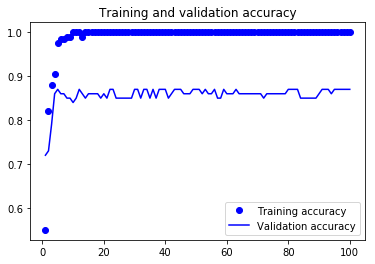Figure 5. Accuracy of the fully connected layers solution.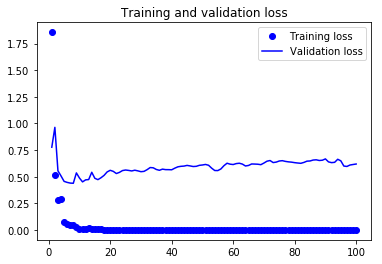Figure 6. Loss of the fully connected layers solution.

Brief discussion of results:

1. Validation accuracy is around 0.85, which is encouraging given the size of the dataset.
2. The model strongly overfits. There’s a big gap between the training and the validation curves.
3. Since we already used dropout, we should increase the size of the dataset to improve the results.

6.3.2. Global average pooling

The difference between this case and the previous one is that, instead of adding a stack of fully-connected layers, we will add a global average pooling layer and feed its output into a sigmoid activated layer.

Note that we are talking about a sigmoid activated layer instead of a softmax one, which is what is recommended by Lin et al. (2013). We are changing to the sigmoid activation because in Keras, to perform binary classification, you should use sigmoid activation and binary_crossentropy as the loss (Chollet 2017). Therefore, it was necessary to do this small modification to the original proposal of Lin et al. (2013).

Code 4 shows the code to build the classifier. Figure 7 and 8 show the resulting learning curves.

Code 4. Global average pooling solution.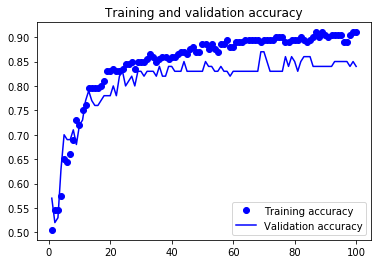Figure 7. Accuracy of the global average pooling solution.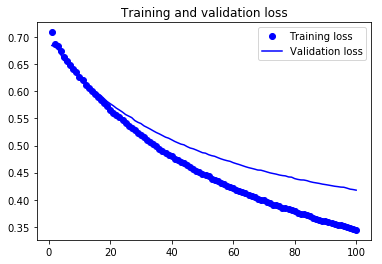Figure 8. Loss of the global average pooling solution.

Brief discussion of results:

1. Validation accuracy is similar to the one resulting from the fully-connected layers solution.
2. The model doesn’t overfit as much as in the previous case.
3. The loss function is still decreasing when the model stops training. Probably, it is possible to improve the model by increasing the number of epochs.

6.3.3 Linear support vector machines

In this case, we will train a linear support vector machines (SVM) classifier on the features extracted by the convolutional base.

To train this classifier, a traditional machine learning approach is preferable. Consequently, we will use k-fold cross-validation to estimate the error of the classifier. Since k-fold cross-validation will be used, we can concatenate the train and the validation sets to enlarge our training data (we keep the test set untouched, as we did in the previous cases). Code 5 shows how data was concatenated.

Code 5. Data concatenation.

Finally, we must be aware that the SVM classifier has one hyperparameter. This hyperparameter is the penalty parameter C of the error term. To optimize the choice of this hyperparameter, we will use exhaustive grid search. Code 6 presents the code used to build this classifier, while Figure 9 illustrates the learning curves.

Code 6. Linear SVM solution.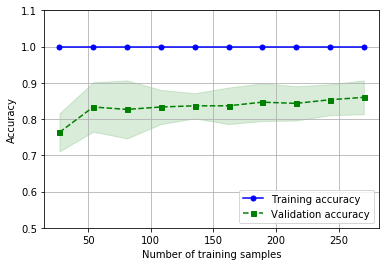Figure 9. Accuracy of the linear SVM solution.

Brief discussion of results:

1. Model’s accuracy is around 0.86, which is similar to the accuracy of the previous solutions.
2. Overfitting is around the corner. Moreover, the training accuracy is always 1.0, which is not usual and can be interpreted as a sign of overfitting.
3. The accuracy of the model should increase with the number of training samples. However, that doesn’t seem to happen. This may be due to overfitting. It would be interesting to see how the model reacts when the dataset increases.

### 7. Summary

• Presented the concepts of transfer learning, convolutional neural networks, and pre-trained models.
• Defined the basic fine-tuning strategies to repurpose a pre-trained model.
• Described a structured approach to decide which fine-tuning strategy should be used, based on the size and similarity of the dataset.
• Introduced three different classifiers that can be used on top of the features extracted from the convolutional base.
• Provided a end-to-end example on image classification for each of the three classifiers presented in this article.

I hope that you feel motivated to start developing your deep learning projects on computer vision. This is a great field of study and new exciting findings are coming out everyday.

I’d be glad to help you, so let me know if you have any questions or improvement suggestions!

### 8. References

1. Bengio, Y., 2009. Learning deep architectures for AI. Foundations and trends in Machine Learning, 2(1), pp.1–127.

2. Canziani, A., Paszke, A. and Culurciello, E., 2016. An analysis of deep neural network models for practical applications. arXiv preprint arXiv:1605.07678.

3. Chollet, F., 2015. Keras.

5. Deng, J., Dong, W., Socher, R., Li, L.J., Li, K. and Fei-Fei, L., 2009, June. Imagenet: A large-scale hierarchical image database. In Computer Vision and Pattern Recognition, 2009. CVPR 2009. IEEE Conference on (pp. 248–255). Ieee.

6. He, K., Zhang, X., Ren, S. and Sun, J., 2016. Deep residual learning for image recognition. In Proceedings of the IEEE conference on computer vision and pattern recognition (pp. 770–778).

7. Krizhevsky, A., Sutskever, I. and Hinton, G.E., 2012. Imagenet classification with deep convolutional neural networks. In Advances in neural information processing systems (pp. 1097–1105).

8. LeCun, Y., Bengio, Y. and Hinton, G., 2015. Deep learning. nature, 521(7553), p.436.

9. Lin, M., Chen, Q. and Yan, S., 2013. Network in network. arXiv preprint arXiv:1312.4400.

10. Pan, S.J. and Yang, Q., 2010. A survey on transfer learning. IEEE Transactions on knowledge and data engineering, 22(10), pp.1345–1359.

11. Rawat, W. and Wang, Z., 2017. Deep convolutional neural networks for image classification: A comprehensive review. Neural computation, 29(9), pp.2352–2449.

12. Simonyan, K. and Zisserman, A., 2014. Very deep convolutional networks for large-scale image recognition. arXiv preprint arXiv:1409.1556.

13. Szegedy, C., Vanhoucke, V., Ioffe, S., Shlens, J. and Wojna, Z., 2016. Rethinking the inception architecture for computer vision. In Proceedings of the IEEE conference on computer vision and pattern recognition (pp. 2818–2826).

14. Tang, Y., 2013. Deep learning using linear support vector machines. arXiv preprint arXiv:1306.0239.

15. Voulodimos, A., Doulamis, N., Doulamis, A. and Protopapadakis, E., 2018. Deep learning for computer vision: A brief review. Computational intelligence and neuroscience, 2018.

16. Yosinski, J., Clune, J., Bengio, Y. and Lipson, H., 2014. How transferable are features in deep neural networks?. In Advances in neural information processing systems (pp. 3320–3328).

17. Zeiler, M.D. and Fergus, R., 2014, September. Visualizing and understanding convolutional networks. In European conference on computer vision (pp. 818–833). Springer, Cham.

### Acknowledgments

Thanks to João Coelho for reading drafts of this.

Bio: Pedro Marcelino is interested in all aspects of machine learning and data analysis. His focus is on data mining & quality, exploratory analysis & visualization, and predictive/prescriptive analytics, but includes testing and benchmarking of different machine learning approaches on real-life problems.

Original. Reposted with permission.

Related:

Top Stories Past 30 Days
Most Popular
Most SharedGet KDnuggets, a leading newsletter on AI, Data Science, and Machine Learning